# GSEB Solutions Class 6 Maths Chapter 3 Playing With Numbers Ex 3.4

Gujarat Board GSEB Textbook Solutions Class 6 Maths Chapter 3 Playing With Numbers Ex 3.4 Textbook Questions and Answers.

## Gujarat Board Textbook Solutions Class 6 Maths Chapter 3 Playing With Numbers Ex 3.4

Question 1.
Find the common factors oft
(a) 20 and 28
(b) 15 and 25
(c) 35 and 50
(d) 56 and 120
Solution:
(a) 20 and 28
Wehave: 20 = 1 x 20
20 = 2 x 10
20 = 4 x 5
All the factors of 20 are: 1, 2, 4, 5, 10 and 20
Again, 28 = 1 x 28
28 = 2 x 14
28 = 7 x 4
AIl the factors of 28 are: 1, 2, 4, 7, 14 and 28 . . .(ii)
From
(i) and
(ii), common factors of 20 and 28 are : 1, 2 and 4.

(b) 15 and 25
Since 15 = 1 x 15
15 = 3 x 5
All the factors of 15 are : 1, 3, 5 and 15 …(i)
Again, 25 = 1 x 25
25 = 5 x 5
All the factors of 25 are: 1, 5 and 25 …(ii)
From
(i) and
(ii), common factors of 15 and 25 are : 1 and 5.

(c) 35 and 50
Since 35 = 1 x 35
35 = 5 x 7
All the factors of 35 are: 1, 5, 7 and 35 ……..(i)
Again, 50 = I x 50
50 = 2 x 25
50 = 5 x 10
All the factors of 50 are: 1, 2, 5, 10, 25, 50 …(ii)
From
(i) and
(ii), common factors of 35 and 50 are : 1 and 5.

(d) 56 and 120
Since 56 = 1 x 56
56 = 2 x 28
56 = 4 x 14
56 = 7 x 8
All the factors of 56 are: 1,2,4, 7, 8, 14,28 and 56.
Again,
120 = 1 x 120
120 = 2 x 60
120 = 3 x 40
120 = 4 x 30
120 = 5 x 24
120 = 6 x 20
120 = 8 x 15
120 = 10 x 12
All the factors of 120 are: 1, 2, 3, 4, 5, 6, 8, 10, 12, 15, 20, 24, 30, 40, 60 and 120
The common factors of 56 and 120 are: 1, 2, 4 and 8.Question 2.
Find the common factors of
(a) 4, 8 and 12
(b) 5, 15 and 25
Solution:
(a) 4, 8 and 12
Factors of 4 are : 1, 2 and 4
Factors of 8 are : 1, 2, 4 and 8
Factors of 12 are : 1, 2, 3, 4, 6 and 12
Common factors of 4, 8 and 12 are: 1, 2 and 4.

(b) 5, 15 and 25
Factors of 5 are : 1 and 5
Factors of 15 are : 1, 3, 5 and 15
Factors of 25 are : 1, 5 and 25
Common factors of 5, 15 and 25 are : 1 and 5.

Question 3.
Find first three common multiples oft
(a) 6 and 8
(b) 12 and 18
Solution:
(a) 6 and 8
Since multiples of 6 are :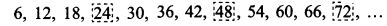Multiples of 8 are :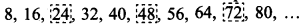The first three common multiples of 6 and 8 are : 24, 48 and 72.

(b) 12 and 18
Since, multiples of 12 are: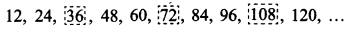Multiples of 18 are :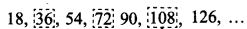First three common multiples of 12 and 18 are : 36, 72 and 108.Question 4.
Write all the numbers less than 100 which are common multiples of 3 and 4.
Solution:
Multiples of 3, which are less than 100, are :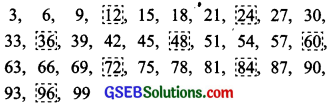Multiples of 4, which are less than 100, are :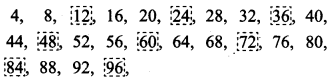Common multiples of 3 and 4, which are less than 100, are :
12, 24, 36, 48, 60, 72, 84 and 96Question 5.
Which of the following numbers are co – prime?
(a) 18 and 35
(b) 15 and 37
(c) 30 and 415
(d) 17 and 68
(e) 216 and 215
(f) 81 and 16
Solution:
(a) 18 and 35
Factors of 18 are : 1, 2, 3, 6, 9 and 18
Factors of 35 are : 1, 5, 7 and 35
Since, their common factor is 1 so
18 and 35 have no common factor except 1.
Thus, 18 and 35 are co-prime numbers.

(b) 15 and 37
Factors of 15 are : 1, 3, 5 and 15
Factors of 37 are : 1, 37
Their common factor is 1, i.e. 15 and 37 do
not have common factors except 1.
Thus, 15 and 37 are co-prime numbers.

(c) 30 and 415
Factors of 30 are: 1, 2, 3, 5, 6, 10, 15 and 30
Factors of 415 are: 1, 5, 83 and 415
Their common factors are: 1 and 5
Thus, 30 and 415 are not co-prime numbers.

(d) 17 and 68
Factors of 17 are: 1 and 17
Factors of 68 are: 1, 2, 4, 17, 34 and 68
Common factors of 17 and 68 are: 1 and 17
Thus, 17 and 68 are not co-prime numbers.

(e) 216 and 215
Factors of 216 are: 1, 2, 3, 4, 6, 8, 9, 12, 18,
24, 27, 36, 54, 72, 108 and 216
Factors of 215 are: 1, 5, 43 and 215
Common factor of 216 and 215 is 1.
Thus, 216 and 215 are co-prime numbers.
(f) 81 and 16
Factors of 81 are: 1, 3, 9, 27 and 81
Factors of 16 are: 1, 2, 4, 8 and 16
Common factors of 81 and 16 is 1.
Thus, 81 and 16 are co-prime numbers.Question 6.
A number is divisible by both 5 and 12. By which other number will that number be always divisible?
Solution:
The given number will be divisible by the product of 5 and 12.
The number will be divisible by 5 x 12 or 60.

Question 7.
A number is divisible by 12. By what other numbers will that number be divisible?
Solution:
The number divisible by 12 will also be divisible by the factors of 12.
Factors of 12 are 1, 2, 3, 4, 6 and 12.
The number will be divisible by 1, 2, 3, 4 and 6.